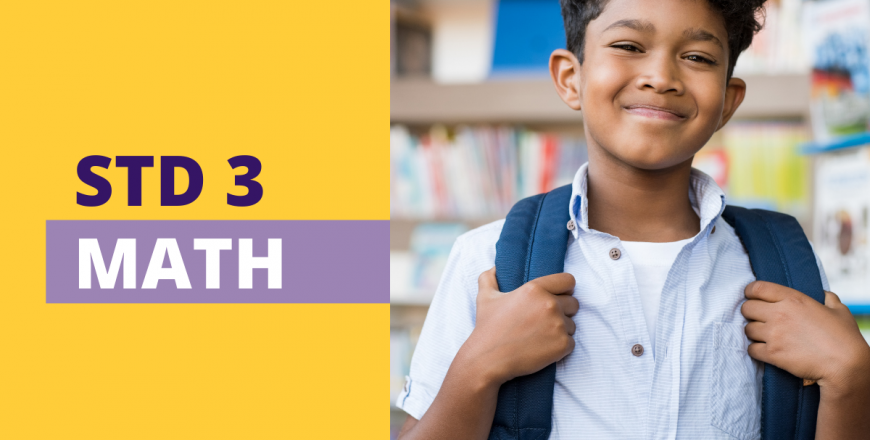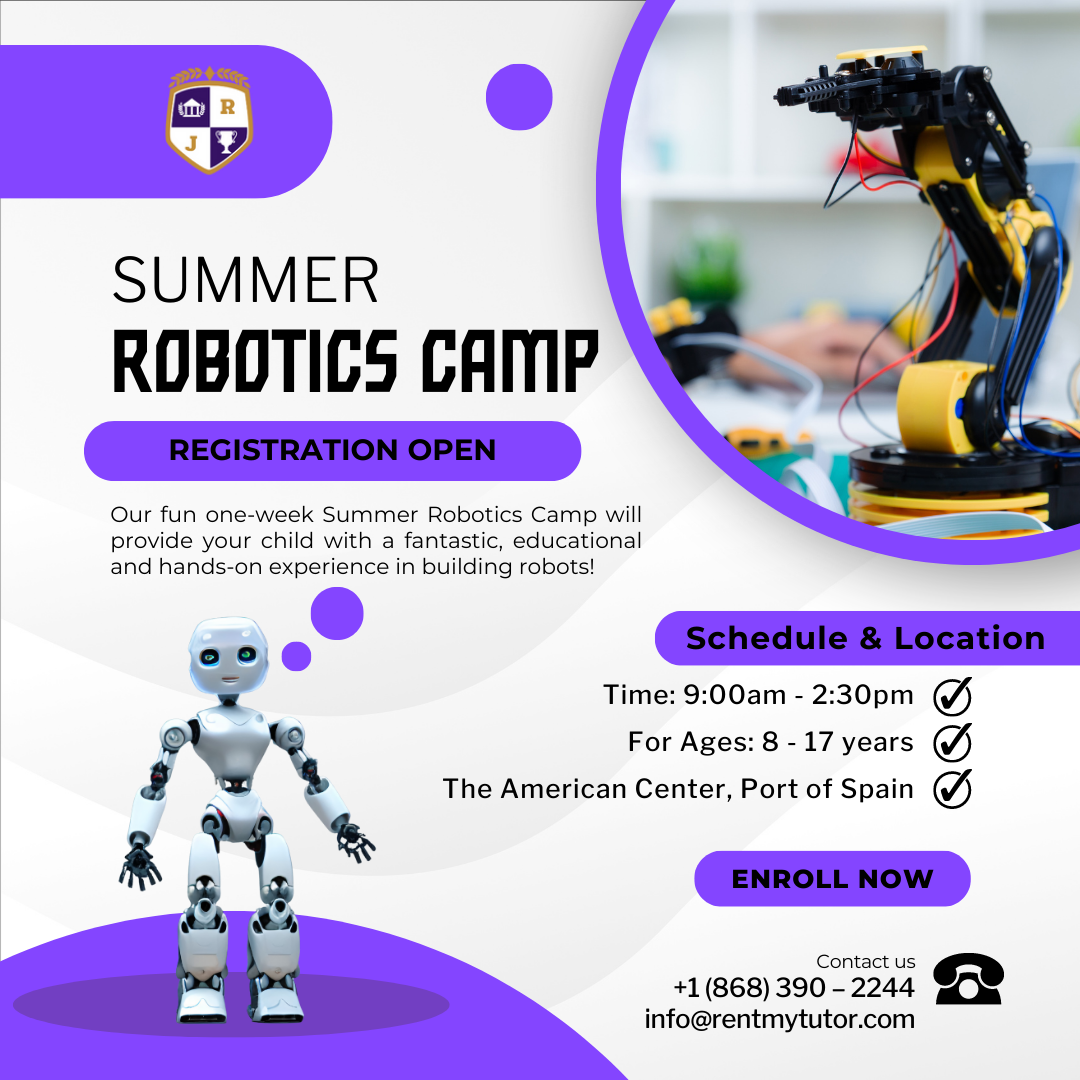# Std. 3 Math

4 out of 5
4
6 reviewsAt Rent My Tutor, we help you connect with the right tutor or class for your needs, right when you need them most. Our innovative Live Learning Platform, personalized approach, and highly credentialed instructors make it easier for you or your student to learn with ease.
One-on-One Tutoring
‐ Live online instruction
‐ Tailored to the students’ needs
\$350/mthly or \$900/Term Membership option
• 1 LIVE session per week (1hr each)
Description:

Class Details

Our One-on_One Classes Include:
• Live course instruction where you’ll meet face-to-face with your eTutor in a virtual classroom
• Interactive and collaborative sessions where learners work on course-specific lessons with their Tutor
• Individual interaction with an expert tutor and an immersive learning experience via Zoom
• After enrolling for your course online, you will create a free Rent My Tutor member account, from which you will be able to access information about your class
• You will have access to superior-class customer service through your account, over the phone or chatbot 7 days a week
NB. You may double or triple your packages accordingly by increasing your online order.

### NUMBER

1
Number Concepts -Std. 3
15

In this lesson, students will learn to:

• Recognize, represent, model, compare and order numbers up to1 000 000 with reference to place value
• Demonstrate an understanding of different types of numbers
• Develop an understanding of rounding to thousands

2
Place Value and Rounding – Std. 3
15

In this lesson, students will learn to:

• Demonstrate an understanding of place value to 9 999
• Compare and order numerals up to 10 000 with reference to place value
• Develop an understanding of rounding to tens, hundreds and thousands

3
Number Patterns – Std. 3
15

In this lesson, students will learn to:

• Recognize and explore number patterns up to 1 000.
• Develop an understanding of different types of numbers by exploring their patterns
• Develop an understanding that pattern recognition can aid in problem solving

4
Number Relationships – Std. 3
10

In this lesson, students will learn to:

• Explore number relationships involving the four operations using number sentences with one unknown

5
Whole Number (Operations): Addition and Subtraction – Std. 3
15

In this lesson, students will learn to:

• Demonstrate an understanding of the algorithm for addition and subtraction
• Solve a variety of word problems using problem solving strategies including mental strategies
• Demonstrate an understanding of estimation skills

6
Whole Number (Operations): Multiplication and Division – Std. 3
25

In this lesson, students will be able to:

• Develop and apply procedures to multiply and divide whole numbers to solve problems
• Solve a variety of word problems using problem solving strategies including mental strategies
• Demonstrate an understanding of estimation skill.
• Use the relationship between multiplication and division to check answers
• Create number stories using appropriate language

7
Mental Mathematics – Std. 3
10

In this lesson, students will be able to:

• Demonstrate skills in mental strategies

8
Fractions – Std. 3
15

In this lesson, students will learn to:

• Develop an understanding of fractions by using concrete, pictorial and symbolic representations
• Demonstrate an understanding of proper and improper fractions and mixed numbers
• Solve problems involving the addition and subtraction of fractions

9
Numerical Language – Std. 3
10

In this lesson, students will learn to:

• Communicate effectively using vocabulary associated with number

### GEOMETRY

1
Solids – Std. 3
10

In this lesson, students will lean to:

• Demonstrate an understanding of the properties of solids and plane shapes
• Solve problems involving solids and plane shapes

2
Plane Shapes – Std. 3
10

In this lesson, students will learn to:

• Demonstrate an understanding of the properties of plane shapes
• Solve problems involving plane shapes

3
Symmetry – Std. 3
10

In this lesson, students will lean to:

• Demonstrate an understanding of angles

4
Geometrical Language – Std. 3
10

In this lesson, students will learn to:

• Communicate effectively using vocabulary associated with geometry

### MEASUREMENT

1
Linear – Std. 3
15

In this lesson, students will learn to:

• Apply measurement principles, including using an instrument, estimation and approximation to solve a wide variety of practical problems.
• Develop a conceptual understanding of a conceptual understanding of perimeter
• Solve problem involving linear measure.

• Apply measurement principles, including using an instrument, estimation and approximation to solve a wide variety of practical problems.
• Develop a conceptual understanding of a conceptual understanding of perimeter
• Solve problem involving linear measure.

2
Mass/Weight – Std. 3
10

In this lesson, students will learn to:

• Demonstrate appropriate techniques when measuring, estimating, and quantifying mass/weight
• Solve problems involving measures of mass/weight
3
Time – Std. 3
20

In this lesson, students will learn to:

• Apply measurement principles, including using an instrument, to solve a wide variety of problems
• Read, interpret and record calendar dates in a variety of formats

4
Capacity – Std. 3
20

In this lesson, students will learn to:

• Demonstrate appropriate techniques when measuring capacity
• Solve problems involving measures of capacity

5
Area – Std. 3
20

In this lesson, students will learn to:

• Demonstrate an understanding of measures of area
• Solve problems involving measures of area

6
Measurement Language – Std. 3
10

In this lesson, students will learn to:

• Communicate effectively using vocabulary associated with measurement.

### STATISTICS

1
Tally Charts and Bar Graphs – Std. 3
15

In this lesson, students will learn to:

• Demonstrate the ability to formulate a problem, collect, organize, represent and interpret data on tally charts and bar graphs (using different scale factors) to make sound decisions and solve problems.
• Demonstrate an understanding about the features of graphs and charts
• Demonstrate an understanding of the methods used to address the problem

2
Statistical Language – Std. 3
10

In this lesson, students will learn to:

• Communicate effectively using vocabulary associated with statistics.

Students who are preparing for Std. 4 or Std. 5 and need additional assistance preparing for the SEA Exams.
4
4 out of 5
6 Ratings

#### Detailed Rating

 Stars 5 3 Stars 4 0 Stars 3 3 Stars 2 0 Stars 1 0

#### {{ review.user }}

{{ review.time }}